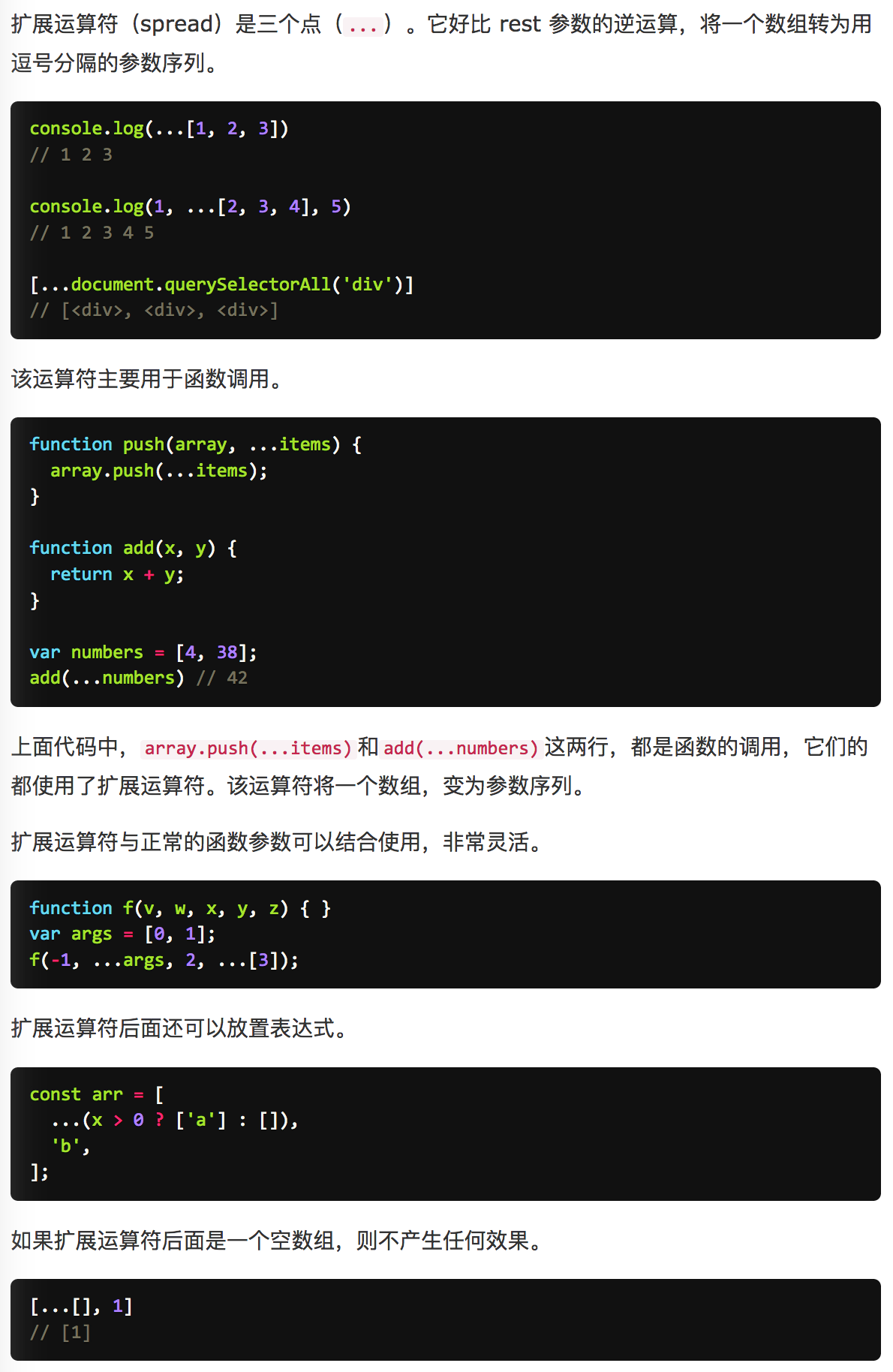# js求最大值

105 篇文章 9 订阅

## Math.max

JavaScript 提供了 Math.max 函数返回一组数中的最大值，用法是：

Math.max([value1[,value2, ...]])

1. 如果有任一参数不能被转换为数值，则结果为 NaN。
2. max 是 Math 的静态方法，所以应该像这样使用：Math.max()，而不是作为 Math 实例的方法 (简单的来说，就是不使用 new )
3. 如果没有参数，则结果为 -Infinity (注意是负无穷大)

1.如果任一参数不能被转换为数值，这就意味着如果参数可以被转换成数字，就是可以进行比较的，比如：

Math.max(true, 0) // 1
Math.max(true, '2', null) // 2
Math.max(1, undefined) // NaN
Math.max(1, {}) // NaN

2.如果没有参数，则结果为 -Infinity，对应的，Math.min 函数，如果没有参数，则结果为 Infinity，所以：

var min = Math.min();
var max = Math.max();
console.log(min > max);

## 原始方法

var arr = [6, 4, 1, 8, 2, 11, 23];

var result = arr;
for (var i = 1; i < arr.length; i++) {
result =  Math.max(result, arr[i]);
}
console.log(result);

## reduce

var arr = [6, 4, 1, 8, 2, 11, 23];

function max(prev, next) {
return Math.max(prev, next);
}
console.log(arr.reduce(max));

## 排序

var arr = [6, 4, 1, 8, 2, 11, 23];

arr.sort(function(a,b){return a - b;});
console.log(arr[arr.length - 1])

## eval

Math.max 支持传多个参数来进行比较，那么我们如何将一个数组转换成参数传进 Math.max 函数呢？eval 便是一种

var arr = [6, 4, 1, 8, 2, 11, 23];

var max = eval("Math.max(" + arr + ")");
console.log(max)

## apply

var arr = [6, 4, 1, 8, 2, 11, 23];
console.log(Math.max.apply(null, arr))

## ES6 ...

var arr = [6, 4, 1, 8, 2, 11, 23];
console.log(Math.max(...arr))

JavaScript专题系列防抖、节流、去重、类型判断、拷贝、最值、扁平、柯里、递归、乱序、排序等，特点是研(chao)究(xi) underscore 和 jQuery 的实现方式。## two:

#### 使用递归函数

var arr = [9,8,55,66,49,68,109,55,33,6,2,1];
var max = arr;
function findMax( i ){
if( i == arr.length ) return max;
if( max < arr[i] ) max = arr[i];
findMax(i+1);
}

findMax(1);
console.log(max);


#### 使用 for 循环遍历

var arr = [9,8,55,66,49,68,109,55,33,6,2,1];
var max = arr;
for(var i = 1; i < arr.length; i++){
if( max < arr[i] ){
max = arr[i];
}
}

console.log(max);


#### 使用apply将数组传入max方法中直接返回

Math.max.apply(null,[9,8,55,66,49,68,109,55,33,6,2,1])


var a=[1,2,3,5];
console.log(Math.max.apply(null, a));//最大值
console.log(Math.min.apply(null, a));//最小值


var a=[1,2,3,[5,6],[1,4,8]];
var ta=a.join(",").split(",");//转化为一维数组
console.log(Math.max.apply(null,ta));//最大值
console.log(Math.min.apply(null,ta));//最小值

02-24224308-251万+
10-25
07-232万+
02-205786
10-201011
01-259353
08-243031
08-041万+
09-053824
08-101056
12-06168
03-066434
04-101102
09-23270点击重新获取扫码支付余额充值